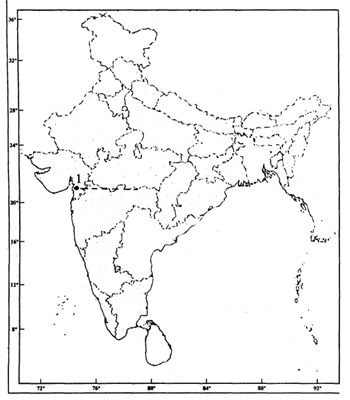# Social Studies - 2007 (Set I - Delhi )

Q. 1. Define the term ‘economic development’. State the two bases of measuring economic development of a country. (1+1=2 marks)

Q. 2. Who is a disabled person ? Give one example each of two types of disabilities. (1+1=2 marks)

Q. 3. Write any four facts about tsunami. (4×½=2 marks)

Q. 4. Explain how Indian industries and trade had been ruined under the British rule in the eighteenth century. (2 marks)

Q. 5. Explain any two main features of Harappan culture. (2×1=2 marks)

Q. 6. Name the northern, eastern, southern and western nlost stations of Golden Quadrilateral express national highway. (4×½=2 marks)

Q. 7. How do the means of mass communication play a vital role in creating awareness among the people ? Explain. (2 marks)

Q. 8. Identify and name the social reformer shown in the picture given below. State any two contributions made by him. (1+1=2 marks)Q. 9. ‘India is a multilingual country’. Justify the statement with two arguments. (2×1=2 marks)

Q. 10. “Wells and tube wells are the most popular means of irrigation in northern plains of India.” Justify the statement with two arguments. (2×1=2 marks)

Q. 11. “Health sector interplays the most significant role in human development.” Justify this statement with two arguments. (2×1=2 marks)

Q. 12. Suggest two facts which should be borne in mind while selecting a site for a building in coastal areas. (2×1=2 marks)

Q. 13. Describeany four characteristics of subsistence farming. (4×1=4 marks)

Or

Describe any four steps taken by the Government to improve Indian agriculture after Independence. (4×1=4 marks)

Q. 14. Describe any four major problems faced by cotton textile industry in India. (4×1=4 marks)

Q. 15. Describe two different approaches by which the number of the poor are estimated in the country. (2+2=4 marks)

Q. 16. “The revolt of 1857 marked a turning point in the history of India.” Support this statement by giving four facts. (2+2=4 marks)

Or

When was the Muslim League formed ? Explain its three main objectives.

Q. 17. “Coal is the main source of energy as well as a raw material for industries in India.”Support this statement with two facts for each. ( 2+2=4 marks)

Q. 18. How does communalism pose a threat to our national unity ? Explain. (1+3=4 marks)

Q. 19. Explain the term ‘Veto’. Name any four countries which have veto power. (4×1=4 marks)

Q. 20. How is disaster managed at different levels ? Explain with one example each, from any four levels. (4×1=4 marks)

Or

Explain any four objectives of a search and rescue team.

Q. 21. When was Indian National Congress founded ? Who played the most important part in its formation ? Describe the main objectives of the Congress. (1+1+4=6 marks)

Or

Describe any six factors that were responsible for the rise of ‘extremism’ in India. 6×1=6 marks)

Q. 22. Why is the conservation of resources essential ? Explain any three measures of conservation of resources. (3+3=6 marks)

Or

“Water is a very important and critical resource in India”. Support this statement by explaining any three points of each. (3+3=6 marks)

Q. 23. Explain with suitable examples the impact of the expansion of business activities in an economy. (6 marks)

Or

Why is the rise of consumer awareness essential ? Explain any four measures to protect consumers’ interest. (2+4=6 marks)

Q. 24. (a) (i) On the given political outline map of India, the place is shown by No. 1, where Congress was split in 1907. Identify the place and name it on the line drawn.

(ii) On the same map shade and name the state where Jallianwala Bagh incident occurred. (1+1=2 marks)

(b) On the given political outline map of India, four geographical features A, B, C and D are marked. Identify these features with the help of the map key and write their correct names on the line marked against each in the map. (4×1=4 marks)

Or

On the given political outline map of India locate and label the following features : (4×1=4 marks)

1. Kanpur - Cotton Textile Centre
2. Kaiga - Nuclear Power Station
3. Haldia - Oil Refinery
4. Kandla - Seaport

Note : The following question is for the Blind Candidates only in lieu of Q. No. 24 (a) and (b). (6×1=6 marks)

1. Name the State where Jallianwala incident occurred.
2. Name the place where Congress was split in 1907.
3. Which is the leading coffee producing state ?
4. Which is the eastern terminal station of ‘East-West Corridor’ ?
5. Name the international air-port located in Gujarat State.
6. Name the Software Technology Park located in Uttar Pradesh.Social Studies 2007 Question Papers Class X